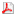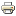Public habilitation talk: RNDr. Dana Černá, Ph.D.Tuesday, March 10, 2020, at 14:00 in M5

#### Wavelet methods for operator equations

Wavelet methods are a useful tool for the numerical solution of various types of operator equations. We introduce the concept of a wavelet basis and two examples of spline wavelet bases.Then we study the wavelet-Galerkin method, namely the existence and uniqueness of the numerical solution and the convergence rate of the method. Furthermore, we also focus on the properties of the resulting systems of linear algebraic equations, especially on their structures and the condition numbers of the discretization matrices. Numerical experiments are provided for integral and integro-differential equations, and as practical application, the jump-diffusion option pricing model is solved.

Last Updated on Wednesday, 04 March 2020 11:31